Mathematical Means and Averages:Generalized Heronian means by Stanislav Sýkora, Extra Byte, Via R.Sanzio 22C, Castano Primo, Italy 20022 in Stan's Library, Ed.S.Sykora, Vol.III. First release July 25, 2009 Permalink via DOI:  10.3247/SL3Math09.002
 Other math articles in Stan's Library | Math Links Math Books | Stan's HUB
 Abstract The classical concept of the Heronian mean of two non-negative real values is subject to a sweeping generalization in order to make it applicable to any subset a of n elements of R+, the set of all positive real numbers. Moreover, it is also extended to include an integer parameter k called rank. The resulting means, denoted as Her(k,a), coincide for n =2 and k = 2 with the classical form. They are shown to satisfy the three basic properties of a mean. Other properties of the new means are also analyzed and compared with other means. In particular, it is shown that Heronian means, despite their apparent complexity, are computationally suprisingly thrifty and efficient. Next article in this series: Generalized Heronian means II.
 1. Introduction In the following we assume that the reader is acquainted with basic definitions and properties  pertinent to means, intended as particular types of mathematical mappings of the sets of n-tuples of real numbers belonging to a domain D onto the domain itself. In the present case, the domain D will be that of all positive real numbers R+, extended later to the set R0+ of all non-negative real numbers. Reference  will be henceforth often linked-to using the format [I, ...]. The classical Heronian mean [2,3], or Hero's mean, is attributed to the Greek mathematician  Hero of Alexandria (10-70 AD) who supposedly introduced it to simplify the formula  for the volume of a truncated pyramid (frustum). The story is described elsewhere ; what interests us here is that it applies to just two positive real numbers a and b. Anticipating the notation to be used later, denote this Heronian mean Her(2,{a,b}). The classical definition then amounts to: (1)It is easy to see that this quantity does satisfy the defining properties of a mean [I, Section 1] except for the invalidating fact that it is limited to doublets of real numbers, rather than generic n-tuples. 2. Generalized Heronian means Let a = {a1,a2,...,an} be an n-tuple of positive real numbers. An obvious way to generalize Eq.(1) is by including inside the parentheses the square roots of all possible products of two elements of a (with repetition) and dividing the whole by the total number of such products, n(n+1)/2. One can write this as: (2)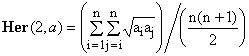What we want to do here is more radical and consists of summing up the k-th roots of all possible distinct products of k elements of a, again with repetition. The number of all such products corresponds to extracting k elements from a bag of n, with replacement, which is C(n+k-1,k), where C(n,m) is the binomial coefficient. This determines the normalization factor and leads to the definition: (3)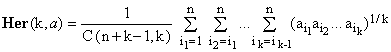Expression (3) defines the [generalized] Heronian mean which we wish to analyze and which can be applied to any n-tuple of non-negative numbers (though, initially, we will consider only the positive ones). The integer parameter k we will call the rank of the Heronian mean. It is interesting to notice that for k = 1, Her(1,a) coincides with the arithmetic mean A(a). Before proceeding further, we must prove that Her(k,a) satisfies the three conditions of a mean [I, Section 1]: m1) Invariance under permutations. Her(k,a) = Her(k,P(a)). Even though the invariance is not immediately transparent from expression (3), it is guaranteed by the way it was set up. The set of all distinct products of total order k of the elements of a is in fact independent of the order of the elements and can not change when they are permuted in any way. Another approach might consist in realizing that i) all the terms in the sum are distinct and ii) any product of k elements of a corresponds to one of the terms. m2) Linear scaling. Her(k,ca) = c Her(k,a). When all elements of a are multiplied by a positive constant c, plain algebra shows that, as required, Eq.(3) is also multiplied by c. m3) Bounds. min(a) ≤ Her(k,a) ≤ max(a). Since, for any i, min(a) ≤ ai, each of the products under the k-th roots is not smaller than min(a)^k. Consequently, none of the summed terms is smaller than min(a), and since the terms are C(n+k-1,k) in number, the expression itself is not smaller than min(a), as required. The same logic of course applies to max(a). Knowing that Her(k,a) is a mean, we can now proceed to analyze its properties. 3. Basic properties of Heronian means Being essentially polynomials in terms of any of the elements of a and, on top of it, polynomials with positive coefficients, it is clear that Heronian means are continuous and strictly monotonous, and therefore strictly regular. A simple numerical counter-example shows that for k > 1 Heronian means are not stable: Let k=2 and a = {1,4}. Then Her(2,a) = 7/3 = 2.333..., but Her(2,{a,7/3}) = 2.319... which is different from 7/3 (even though the difference is small). Another question is whether the Heronian means are simple, i.e., whether the equation Her(k,{a,x}) = Her(k,a) has always a unique solution for x. We know [I, Arithmetic mean proofs] that this is true for k = 1. From the strict monotonicity and continuity it is evident that if the solution exists, it is unique. The real question is therefore whether it always exists. This can be answered positively in virtue of the following reasoning: When x = 0, Her(k,{a,0}) contains exactly the same non-zero summation terms (see Eq.3) as Her(k,a) but, since the number of elements is (n+1) instead of n, we have Her(k,{a,0}) = [C(n+k-1,k)/C(n+k,k)] Her(k,a) = [n/(n+k)] Her(k,a). Since k > 0, this implies that Her(k,{a,0}) < Her(k,a). For x growing to infinity, instead, Her(k,{a,x}) grows to infinity as well and, consequently, there exists a value of x = X for which Her(k,{a,X}) > Her(k,a). The continuity of Her(k,{a,x}) then guarantees that there indeed exists an x in the interval (0,X) at which the two expressions are equal. We therefore conclude that all Heronian means are simple but, for k > 1, they are not stable. It is also easy to see that, for k > 1, Heronian means are not coherent, i.e., given two sets a and b of n positive real numbers, the mean Her(k,{a,b}) is not identically equal to Her(k,{Her(k,a),Her(k,b)}). To show this, use a simple counterexample with k = 2, and a={1,4} and b={1,9}. One has Her(2,a) = 7/3, Her(2,b) = 13/3, but Her(2,{7/3,13/3}) = 3.282 ... which differs from the mean of the combined set Her(2,{1,1,4,9}) = 3.200 (even though again relatively little). 4. Computability properties of Heronian means Despite the somewhat forbidding nature of the definition (3), it turns out that Heronian means are in practice reasonably thrifty and efficient. Apart from the counter of input elements, only k registers are needed to compute Her(k,a) and every element takes one generic power, k multiplications and k additions (in addition, there is the final normalization which requires a cycle of k multiplications and k divisions). For k > 1 this is certainly much more taxing than the arithmetic mean but, for moderate k values, not particularly bad (better than median or robust means). The algorithm uses k real-valued registers s1,s2,...,sk. We will first write down the algorithm before discussing how it works: First, initialize the algorithm by resetting all the registers si to zero. For each input value aj, do: Set a variable z equal to (aj)^(1/k) Set s1 equal to s1 + z For each consecutive si, set si equal to si + z*si-1 (this is a cycle of (k-1) steps) Note that in each step, the si-1 value is the one which has been just modified in the preceding step. Repeat (2) for all the n input values aj Compute the binomial coefficient C(n+k-l,k) using any standard method The result is Her(k,a) = sk/C(n+k-l,k) The way the algorithm operates is best understood considering a special case such as k = 3. As a shorthand, we set zj = (aj)^(1/k). After the first value, a1, has been input, we have:    s1 = z1    s2 = z1z1    s3 = z1z1z1    n = 1 : normalization C(3,3) = 1 After inputting the second value the rank is still higher than the number od input elements. We have:    s1 = z1 + z2    s2 = z1z1 + z1z2 + z2z2    s3 = z1z1z1 + z1z1z2 + z1z2z2 + z2z2z2    n = 2 : normalization C(4,3) = 4 With the third input value the number of input elements equals the rank:    s1 = z1 + z2 + z3    s2 = z1z1 + z1z2 + z2z2 + z1z3 + z2z3 + z3z3    s3 = z1z1z1 + z1z1z2 + z1z2z2 + z2z2z2 + z1z1z3 + z1z2z3 + z2z2z3 + z1z3z3 + z2z3z3 + z3z3z3    n = 3 : normalization C(5,3) = 10 The fourth input value brings the number of input elements above the rank. The result is:    s1 = z1 + z2 + z3 + z4    s2 = z1z1 + z1z2 + z2z2 + z1z3 + z2z3 + z3z3 + z1z4 + z2z4 + z3z4 + z4z4    s3 = z1z1z1 + z1z1z2 + z1z2z2 + z2z2z2 + z1z1z3 + z1z2z3 + z2z2z3 + z1z3z3 + z2z3z3 + z3z3z3        + z1z1z4 + z1z2z4 + z2z2z4 + z1z3z4 + z2z3z4 + z3z3z4 + z1z4z4 + z2z4z4 + z3z4z4 + z4z4z4    n = 4 : normalization C(6,3) = 20 It is now evident how in each case the individual terms are formed and how each distinct product of a given rank arises once and only once. In this way, it is surprisingly easy to evaluate the Heronian means of even very high ranks. There does not appear to be any way to derive a formula which would combine the Heronian means of a given rank and come out with the Heronian mean of the same rank of the combined set. Consequently, unless such a formula is discovered, Heronian means of rank k > 1 are not wrappers. 5. Alternative expressions for Heronian means Let us write any decomposition of the integer number k > 0 into n non-negative integers ei (i.e., allowing 0) as (4)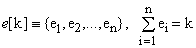We will also use the following shorthand notations (5)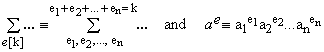for the sum over all distinct decompositions of k into n terms and for the "power" of the set a to the decomposition e, respectively. Using these conventions, Eq.(3) can be now rewritten as (6)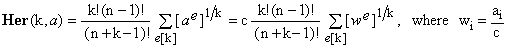In virtue of the property (m2), the constant c in the second form can be any positive real number. Notice that setting c > max(a) or, alternatively, c < min(a), one can make all the wi values smaller than 1 or, respectively, greater than 1 - a feature which might come handy when discussing the properties of Her(k,a). Since, within the formula, k is fixed, one can further simplify the latter equations by introducing a still another set of auxiliary values zi as follows: (7)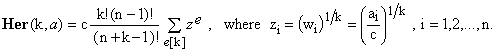Finally, one can easily show the validity of the following analytical formula: (8)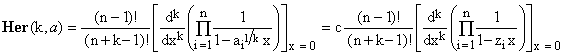To prove Eq.(8), consider the following function F(x;z) of a variable x for some given set of real numbers z: (9)Here the first form is the definition of F(x;z), the second results by expanding each of the product terms into a series in x, and the third by collecting together all terms of the final product with the same power p of x. Notice that all the series expansions, and therefore also the function F(x;z), exist and are totally continuous in x whenever |x| < 1/max(z). Consequently, at x = 0, the derivatives of any order of the function F(x;z) exist and it is evident that (10)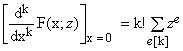, making the rest of the proof is elementary. Notice the Eq.(8) illustrates nicely the invariance of the generalized Heronian mean under permutations of the elements of a, guaranteed in this form simply by the commutativity of the real numbers product. 6. A graphical example of Heronian means It is instructive to plot a certain number of the Heronian means of various ranks for a fixed sample set, together with the classical means, in the same way adopted in [I, Fig.1]. Figure 1. Heronian means (yellow dots) Her(k;a) as functions of k for the set a = {1,2,3,4,5,6,7,8,9,10}.The thin black curve traces the Hölder path H(p,a). The horizontal lines indicate, for the same set a, the minimum, the harmonic mean H, the geometric mean G, the arithmetic mean A, the quadratic mean Q and the maximum. The graph on the right extends to much higher values of k and expands the vertical interval comprised between the geometric and the arithmetic means.   7. Some conjectures Several features of Figure 1 are notable. In particular, it appears that:   a) Her(k,a) decreases with increasing rank k.   b) For any k, G(a) < Her(k,a) ≤ A(a), where G and A are the geometric and arithmetic means, respectively.   c) When k grows to infinity, Her(k,a) rapidly approaches a limit which is greater than G(a). The question now is whether we can prove these conjectures in a rigorous way. They are more than just intuitions since they persist when Monte-Carlo like numeric simulations are carried out with different, randomly chosen sets a of positive real numbers (several million combinations were tested). We will return to these conjectures in a separate article. For now, let us point out that, if proved, they might have a considerable value as a source of novel inequalities. Moreover the limit Her(a) = limk→∞ Her(k,a), provided that it really exists for every set a, has all the properties of a mean. This is due to the fact that the any limit transition on a parametric family of means preserves the conditions m1, m2,and m3. The limit of Her(k,a) therefore represents a new construct, to be called Hermean Her(a). 8. Conclusions It was shown that the classical Heronian mean of two non-negative real numbers can be generalized to give rise to a parametrized family of generalized Heronian means Her(k,a), where a is any n-tuple of real numbers. For k = 1, this coincides with the arithmetic mean, while the classical Heronian mean corresponds to k = 2 and n = 2. Though the generalized Heronian means lack some desirable properties, most notably stability and coherence, they do possess others, such as strong regularity and simplicity. Moreover they are numerically quite thrifty and efficient and therefore suitable for practical applications. Numeric tests show that the family probably satisfies a notable inequality, namely A(a) ≥ Her(k,a) ≥ Her(k+1,a) ≥ G(a) where, as usual, A(a) and G(a) denote the arithmetic and the geometric means, respectively. Likewise hypothetical, but highly likely, is the existence of the limit mean limk→∞Her(k,a) = Her(a) which satisfies A(a) ≥ Her(a) ≥ G(a), with the equalities valid if and only if all the elements of a are the same. Further investigation of these conjectures is underway.
 References and Links Sykora S., Mathematical Means and Averages: Basic Properties, Stan's Library, Ed.S.Sykora, Vol.III, 2009. an introductory reference text for this article. Bullen P.S., Mitrinovic D.S., Vasic M., Means and Their Inequalities, Springer 1999. ISBN 9-027-72629-9. more >> Heronian mean on Wikipedia and MathWorld. Hero of Alexandria. Weisstein E.W., Pyramidal Frustum at MathWorld. Eves H., Great Moments in Mathematics Before 1650, Mathematical Association of America 1983. ISBN 978-0883853108. more >> Mathematical Means and Averages: Generalized Heronian means, URL of this article.  Added on 13 October 2009: Generalized Heronian means II, Stan's Library, Ed.S.Sykora, Vol.III, 2009. Proofs of Heronian means inequalities for the special cases of k = 1,2 (any n) and n = 2 (any k).
 TOP | Other math articles in Stan's Library | Math Links Math Books | Stan's HUB |